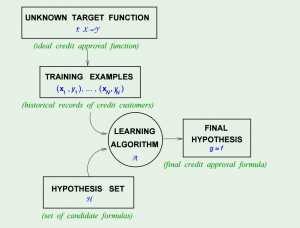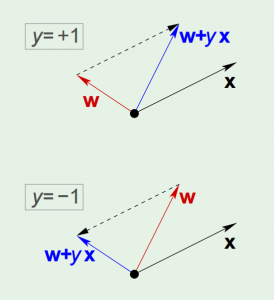# Learning from Data 1: The Learning Problem

Learning from Data is a Machine Learning MOOC taught by Caltech Professor Yaser Abu-Mostafa. I took the course in 2014 through edX. It was a great course, well-organized and into the details.

Recently I have been reviewing the notes and the summary of the course, and I thought I might just post them here. All my code used for the homework can be found at https://github.com/Chaoping/Learning-From-Data.

Lecture 1: The Learning Problem

Lecture 1 is the brief introduction of the course. It answered the question of “what is learning”. So the essence of learning is that:

• A pattern exists, which we are trying to find
• We cannot pin it down mathematically (otherwise we would not need to “learn”)
• We have data

And a learning problem can be formalized as:

• Input: $\mathbf{x}$
• Output: $y$
• Target function: $f: X \rightarrow Y$, which we do not know
• Data: $(\mathbf{x}_1, y_1), (\mathbf{x}_2,y_2),…,(\mathbf{x}_N,y_N)$ $$\downarrow$$
• Hypothesis: $g: X \rightarrow Y$, to approximate the target function.

In fact, to come up with the final hypothesis $g$, we are using a hypothesis set $H$, in which there are infinite hypotheses. A chart in the course slides shows the concept:The Hypothesis Set $H$ and the Learning Algorithm $A$ together are referred as the “learning model”.

Then the simplest example of learning is the “perceptron”, its linear formula $h \in H$ can be written as: $$h(x) = \mathrm{sign} ((\sum_{i=1}^{d}{w_i}{x_i})-t)$$ where t represents the threshold.

If we change the notation of $t$ to $w_0$, and introduce an artificial coordinate $x_0 = 1$, all of a sudden it can now be written as: $$h(x) = \mathrm{sign}(\sum_{i=0}^{d}{w_i}{x_i})$$ or equivalently, $$h(x) = \mathrm{sign}(\mathbf{w}^\intercal\mathbf{x})$$

The concept of perceptron’s learning algorithm is rather intuitive, that it tries to correct a random misclassified point every iteration, by: $$\mathbf{w} = \mathbf{w} + {y_n}\mathbf{x_n}$$ The correction can be visualized as the following:The algorithm keeps iterating, and eventually it will come to a point where all points are correctly classified, so long as the data is linearly-separable.

There are 3 types of learning:

• Supervised Learning: input, output
• Unsupervised Learning: input, no output
• Reinforcement Learning: input, grade for output## Author: Chaoping Guo

Learning is a privilege that I am grateful for.